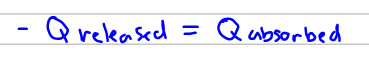# Problem: A 13.0 g sample of granite initially at 82.0 oC is immersed into 26.0 g of water initially at 23.0 oC. What is the final temperature of both substances when they reach thermal equilibrium? (For water, Cp =4.18 J/g•oC and for granite, Cp =0.790 J/g•oC.)

###### FREE Expert Solution

First, we have to know that the heat released by the granite is equal to the heat absorbed by water.Q released is negative because of the direction of the heat.

Next, we can use the equation we use for calorimetry:

96% (436 ratings)###### Problem Details

A 13.0 g sample of granite initially at 82.0 oC is immersed into 26.0 g of water initially at 23.0 oC. What is the final temperature of both substances when they reach thermal equilibrium? (For water, Cp =4.18 J/goC and for granite, Cp =0.790 J/goC.)